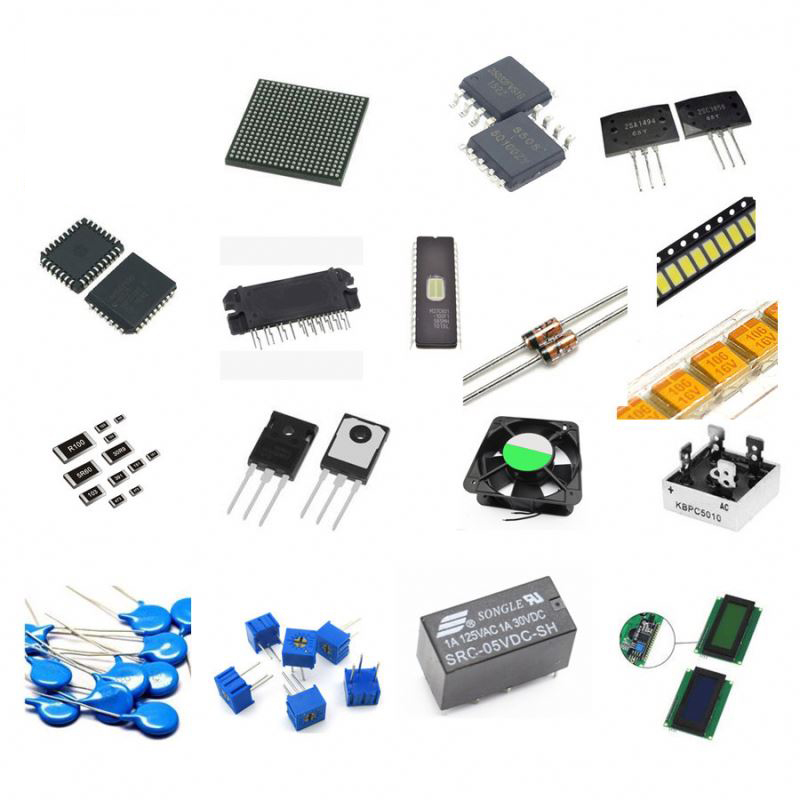< img src="https://mc.yandex.ru/watch/53493796" style="position:absolute; left:-9999px;" alt="" />
Need Help?## Hot selling Electronic ic AD7111ABRZ

• Type: integrated circuit
• Brand Name: Famous
• D/C: 20+
• Description: IC Chip
• Voltage – Breakdown: Standard
• Frequency – Switching: Standard
• Power (Watts): Standard
• Operating Temperature: Standard
• Mounting Type: Standard
• Voltage – Supply (Min): Standard
• Voltage – Supply (Max): Standard
• Voltage – Output: Standard
• Current – Output / Channel: Standard
• Frequency: Standard
• Current – Output (Max): Standard
• Current – Supply: Standard
• Voltage – Supply: Standard
• Frequency – Max: Standard
• Power – Max: Standard
• Voltage Supply – Internal: Standard
• Frequency – Cutoff or Center: Standard
• Current – Leakage (IS(off)) (Max): Standard
• Current – Output High, Low: Standard
• Current – Peak Output: Standard
• Voltage – Forward (Vf) (Typ): Standard
• Current – DC Forward (If) (Max): Standard
• Current Transfer Ratio (Min): Standard
• Current Transfer Ratio (Max): Standard
• Voltage – Output (Max): Standard
• Voltage – Off State: Standard

## Description

• Type: integrated circuit
• Brand Name: Famous
• D/C: 20+
• Description: IC Chip
• Voltage – Breakdown: Standard
• Frequency – Switching: Standard
• Power (Watts): Standard
• Operating Temperature: Standard
• Mounting Type: Standard
• Voltage – Supply (Min): Standard
• Voltage – Supply (Max): Standard
• Voltage – Output: Standard
• Current – Output / Channel: Standard
• Frequency: Standard
• FET Type: Standard
• Current – Output (Max): Standard
• Current – Supply: Standard
• Voltage – Supply: Standard
• Frequency – Max: Standard
• Power – Max: Standard
• Tolerance: Standard
• Voltage Supply – Internal: Standard
• Frequency – Cutoff or Center: Standard
• Current – Leakage (IS(off)) (Max): Standard
• Isolated Power: Standard
• Voltage – Isolation: Standard
• Current – Output High, Low: Standard
• Current – Peak Output: Standard
• Voltage – Forward (Vf) (Typ): Standard
• Current – DC Forward (If) (Max): Standard
• Input Type: Standard
• Output Type: Standard
• Current Transfer Ratio (Min): Standard
• Current Transfer Ratio (Max): Standard
• Voltage – Output (Max): Standard
• Voltage – Off State: Standard
• Static dV/dt (Min): Standard

SEND EMAIL TO US